Learning Library

Math Facts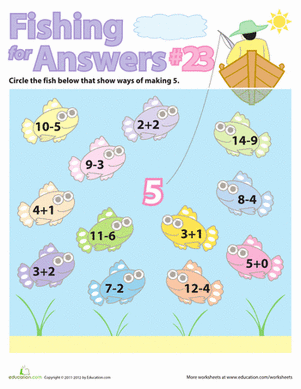Fishing For Math Facts: Five

Make math fun with this series of "Fishing for Answers" math facts!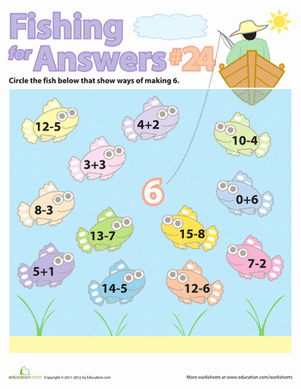Fishing For Math Facts: Six

Give your child a great game for practicing math facts! She'll "fish" for the right answer by solving all the simple subtraction and addition equations.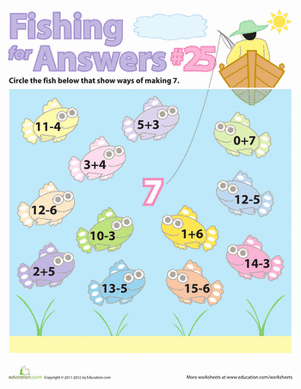Fishing For Math Facts: Seven

Go fishing for math facts with a fun game! Your first grader will practice solving simple addition and subtraction problems.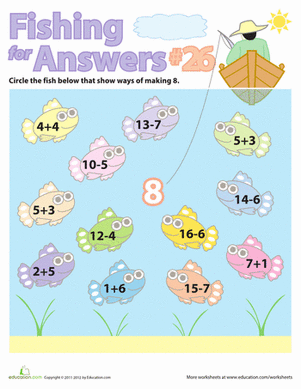Fishing For Math Facts: Eight

Make math fun with this series of "Fishing for Answers" addition and subtraction math facts.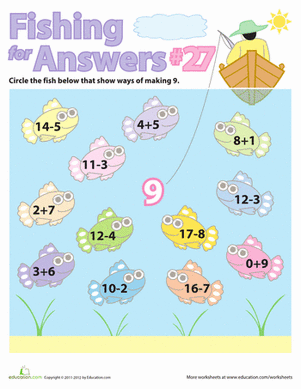Fishing For Math Facts: Nine

Give your first grader a great visual learning game, where he can "Fish for Answers" and learn his math facts!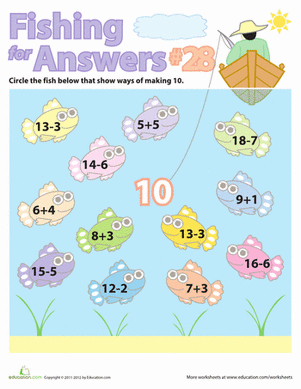Fishing For Math Facts: Ten

Math got your child down in the dumps? Just go fishing! Play the "Fish for Answers" game to learn simple addition and subtraction.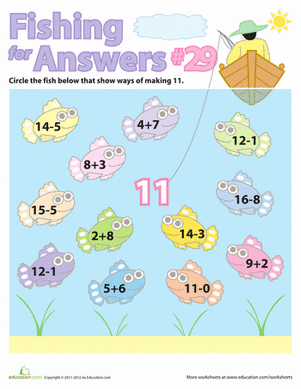Fishing For Math Facts: Eleven

Make math facts fun with this series of "Fishing for Answers" addition and subtraction games!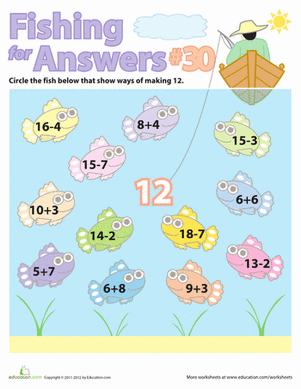Fishing For Math Facts: Twelve

How many different ways can you add and subtract to make the number twelve? Find out with a fun "Fishing for Answers" math facts game!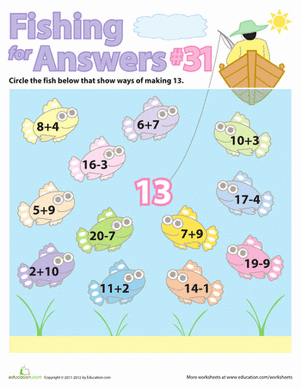Fishing For Math Facts: Thirteen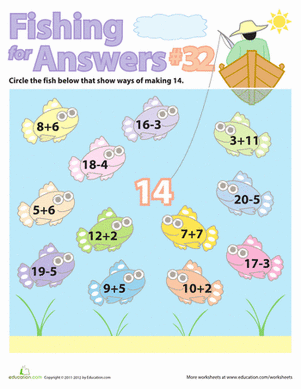Fishing For Math Facts: Fourteen

Make math fun with this series of "Fishing for Answers" math facts. Your child will be able to practice doing simple subtraction and addition equations.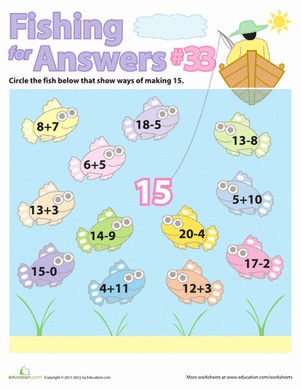Fishing For Math Facts: Fifteen

Does your child need a jump-start on simple arithmetic? Give her a great game to get started learning addition and subtraction math facts!

Create new collection

0

New Collection>

0 items

What could we do to improve Education.com?